Formalism.

The real problem with landscape and almost everything is that once you are in the woods you don’t have to think about the trees any more.  You have to think about something else.  You have to think about some kind of abstractions, some kind of control that you’re gonna put on the trees.  And if you think of the trees you’re not going to think about the abstraction.  You’ve got do something else beside be there, cos being there just doesn’t do it.

Jan Groover

I hope you find something that you like on this photo-blog.  These images show things that stop me, make me look, admire, connect, wonder and to sometimes just simply question what is or what might be going on.

Please also keep an eye on Adam Dunning's blog and also the galleries on his website.  Recent work includes his 'Beside the Seaside, Beside the Sea' gallery which has received very favourable reviews and his very latest gallery Some birds, pigeons mostly, and a squirrel.  Adam's blog posts also feature some wonderful images from around Scarborough, Whitby, Filey, Bempton and Cayton plus Sunk Island on the north bank of the River Humber.  The latest images are from North and South Bay, Scarborough and with others from Germany.  These include some very interesting responses to the Covid19 pandemic.

Mick Fattorini will be exhibiting his latest work very soon in Leicester although this depends on the Covid lockdown conditions.  See here his website.

Sciryuda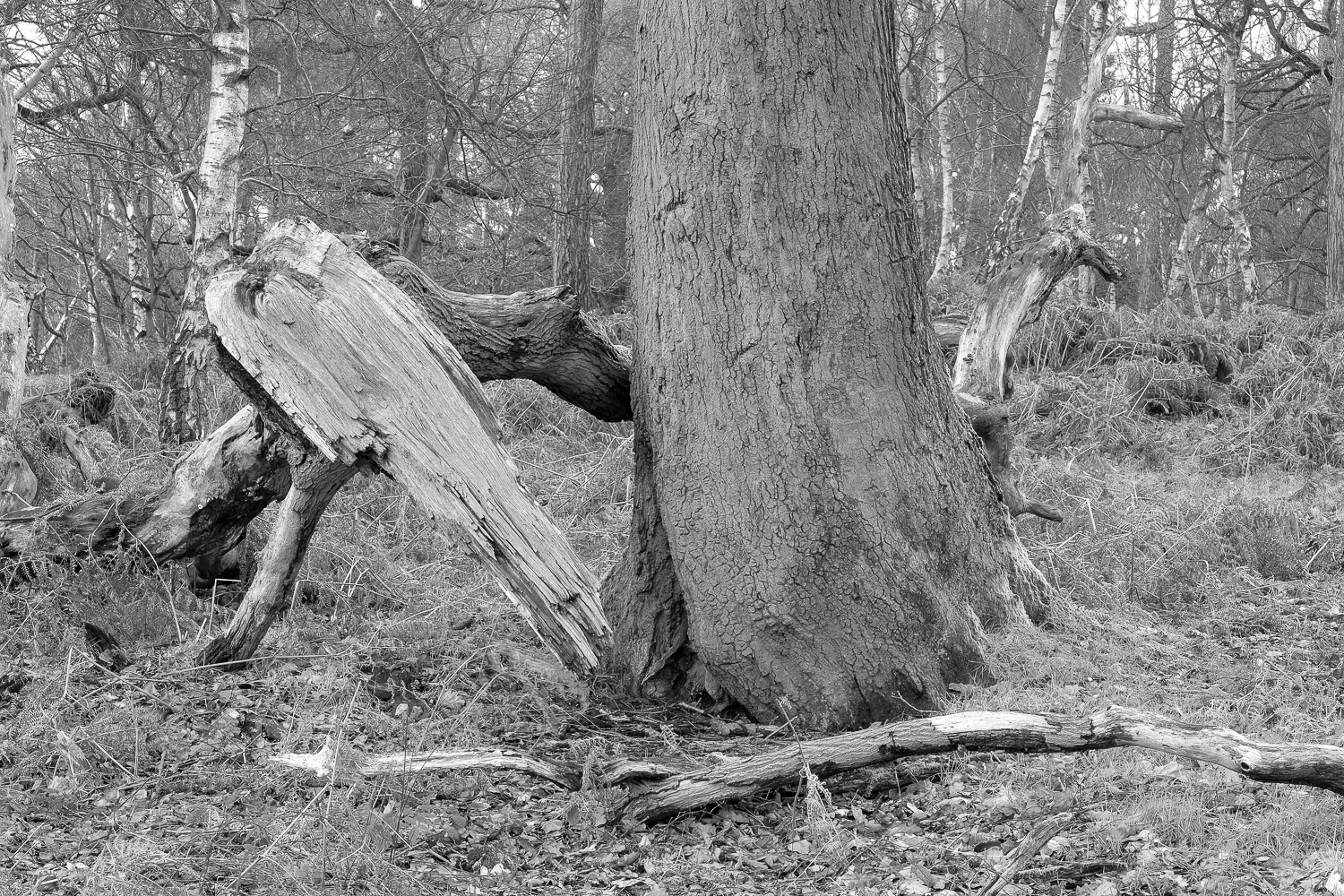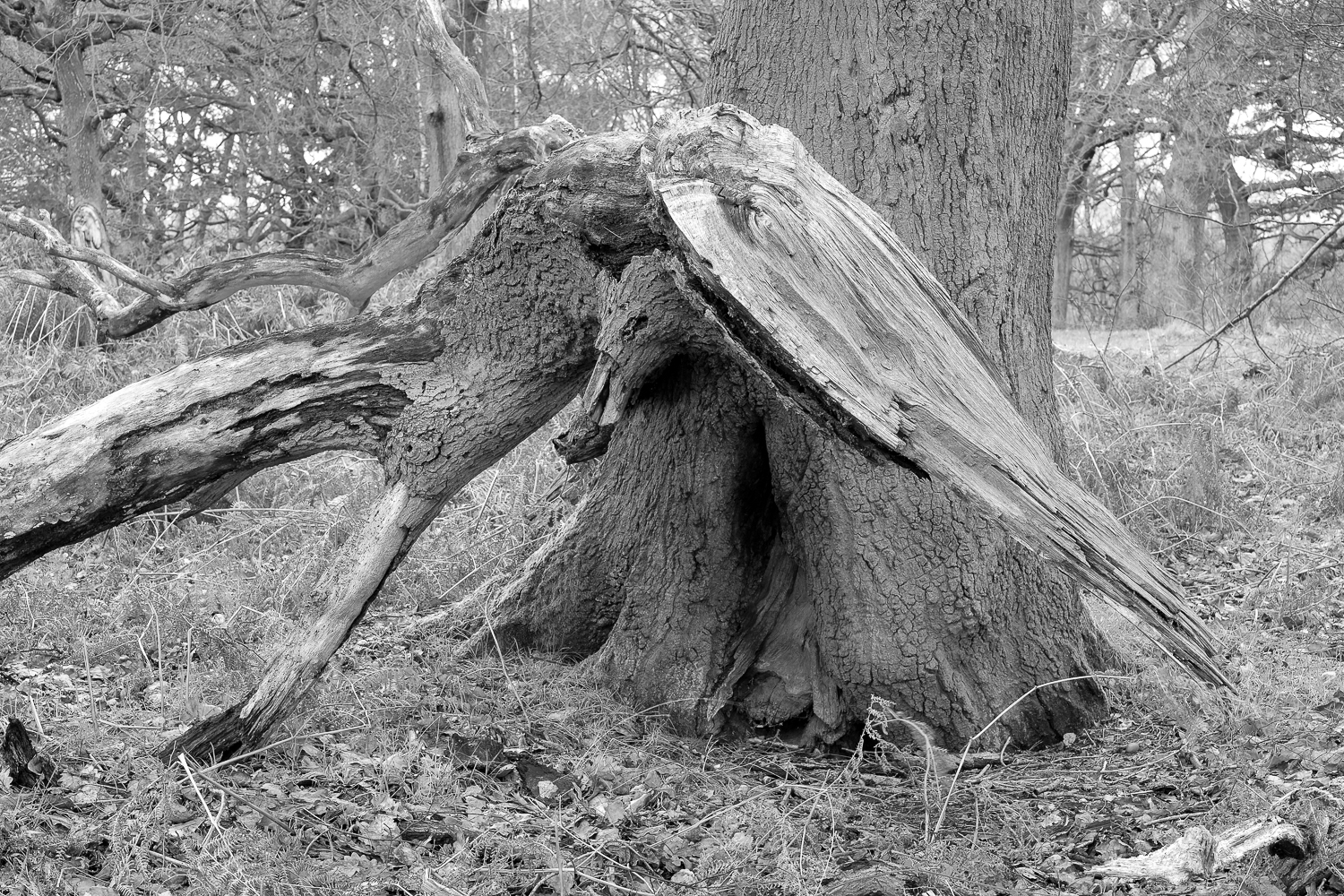S e a r c h i n g.   O a k.   C r o w n   H i l l   P l a n t a t i o n.

S c i r y u d a.   S h e r w o o d   F o r e s t,   N o t t i n g h a m s h i r e.

SciryudaS t o r m.   B u d b y   S o u t h   F o r e s t.

S c i r y u d a.   S h e r w o o d   F o r e s t,   N o t t i n g h a m s h i r e.

Sciryuda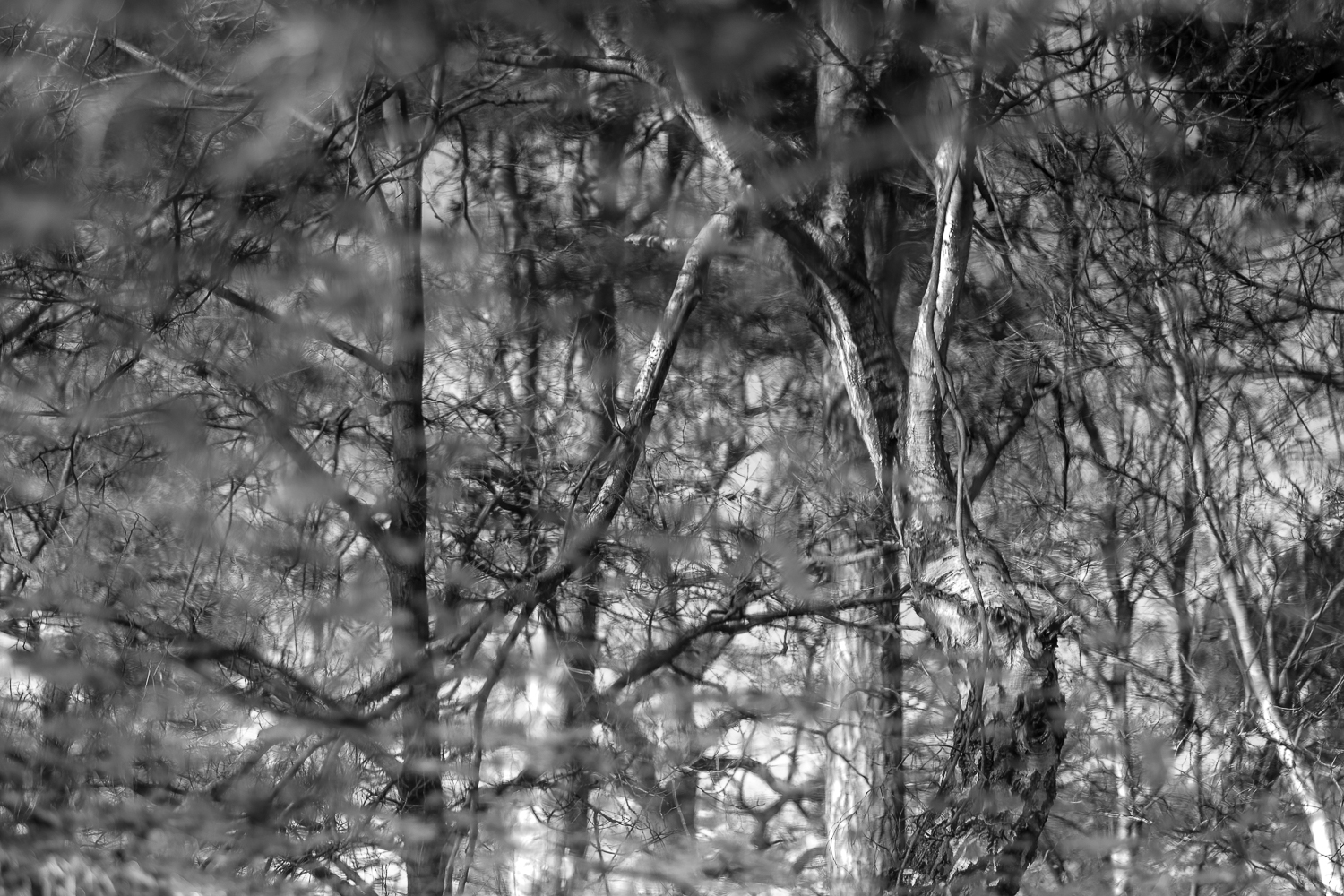B i r c h,   O a k,   P i n e.  W i l d   P o n d.

C r o w n   H i l l   P l a n t a t i o n,   B u d b y   S o u t h   F o r e s t

S c i r y u d a,   S h e r w o o d   F o r e s t,   N o t t i n g h a m s h i r e.

Sciryuda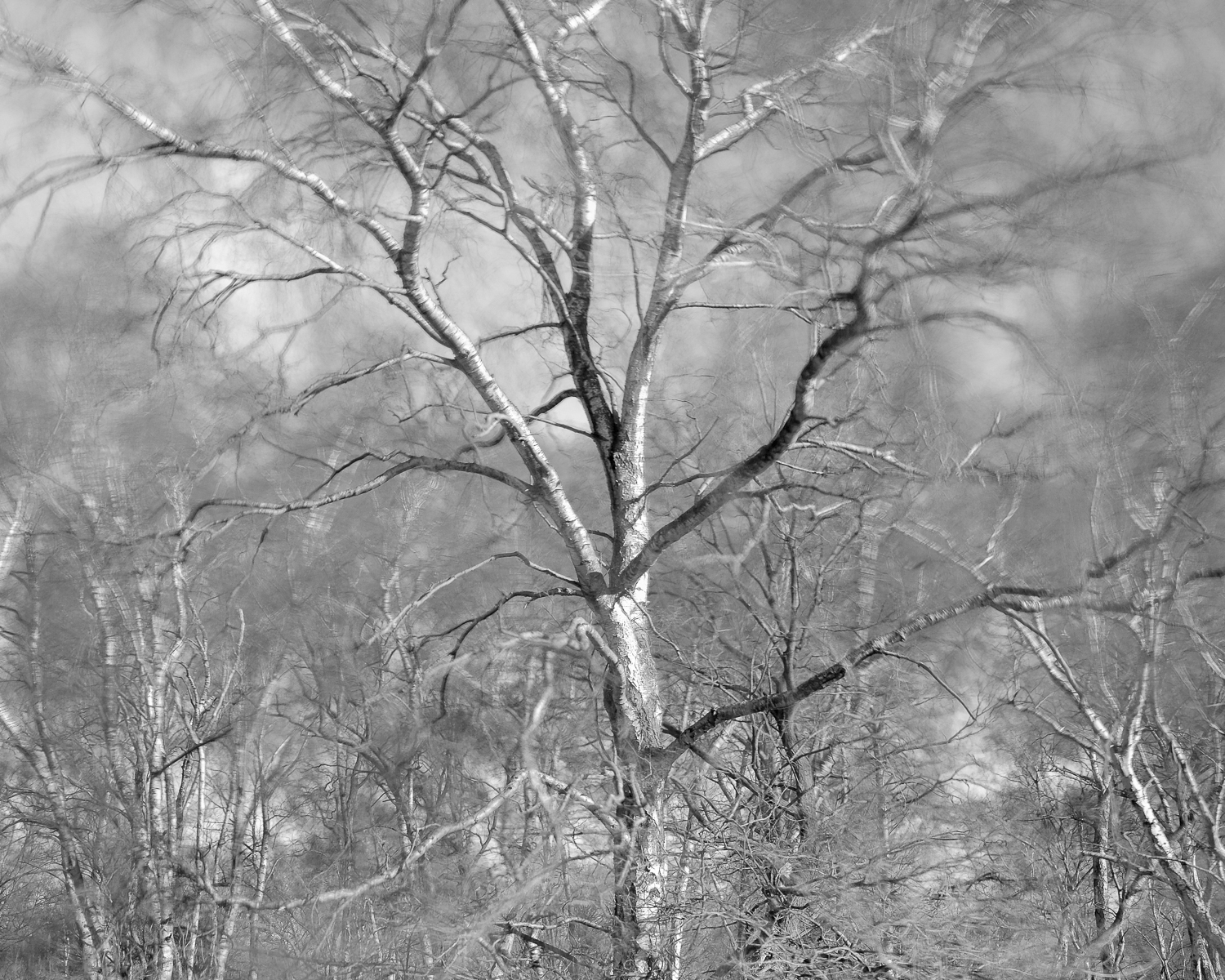S i l v e r   B i r c h   -   ( S t o r m   C i a r a ).   B u r s t h e a r t   H i l l.

S c i r y u d a.   S h e r w o o d   F o r e s t,   N o t t i n g h a m s h i r e.

Sciryuda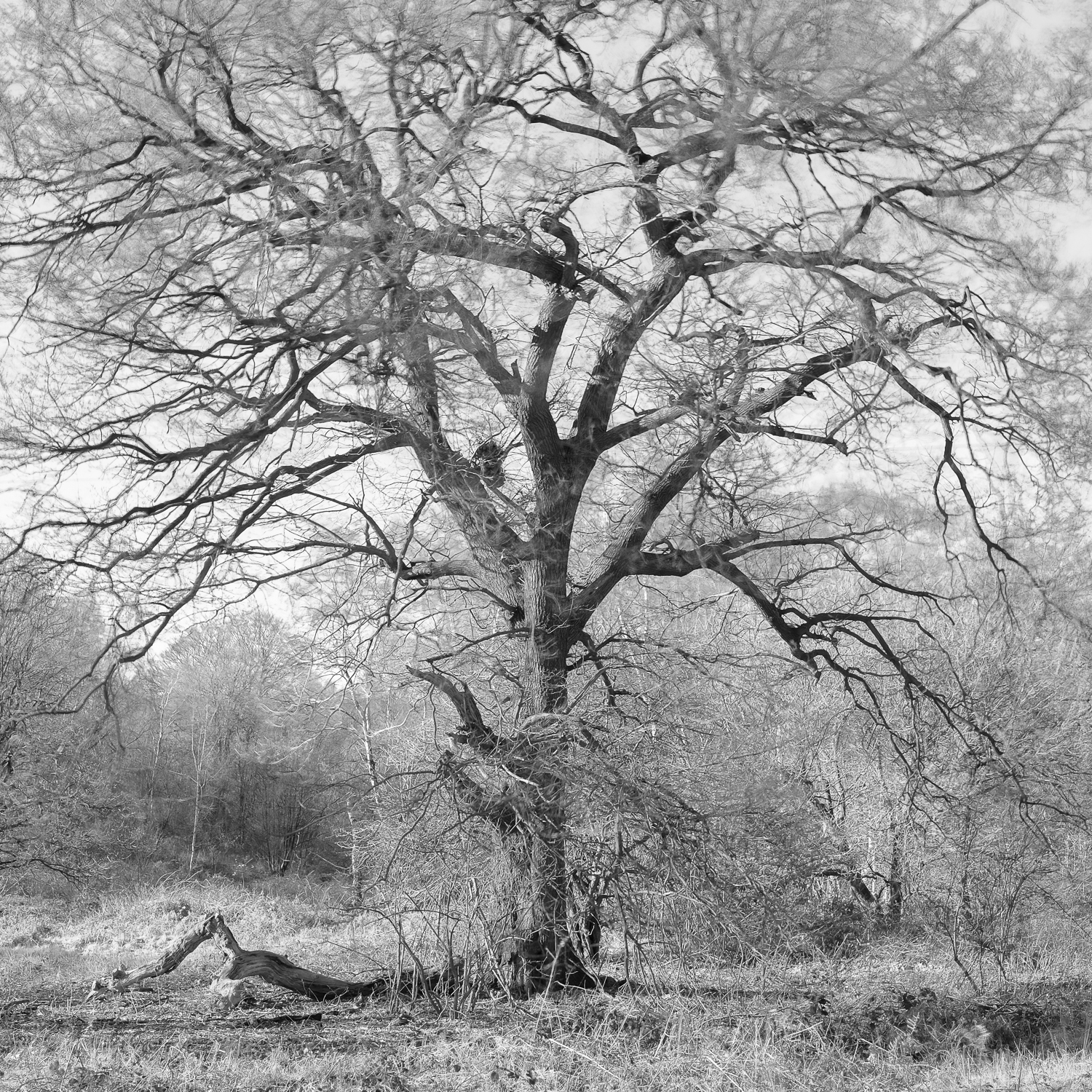O a k.   N e a r   B u r s t h e a r t   H i l l.

S c i r y u d a,   S h e r w o o d   F o r e s t,   N o t t i n g h a m s h i r e.

Sciryuda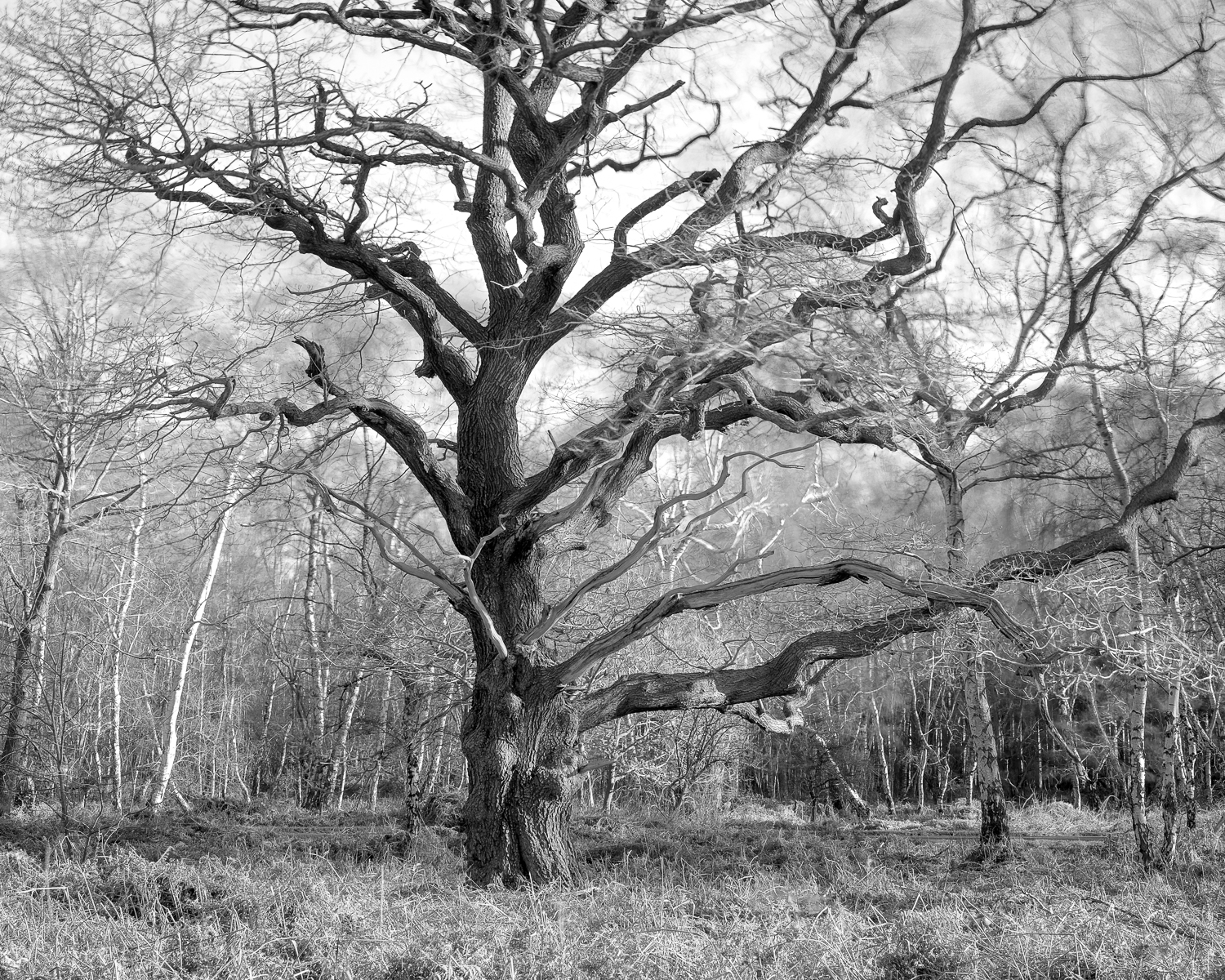O a k.   N e a r   t o   B u r s t h e a r t   H i l l   -   S h e r w o o d   H e a t h

S c i r y u d a,   S h e r w o o d   F o r e s t,   N o t t i n g h a m s h i r e.

Sciryuda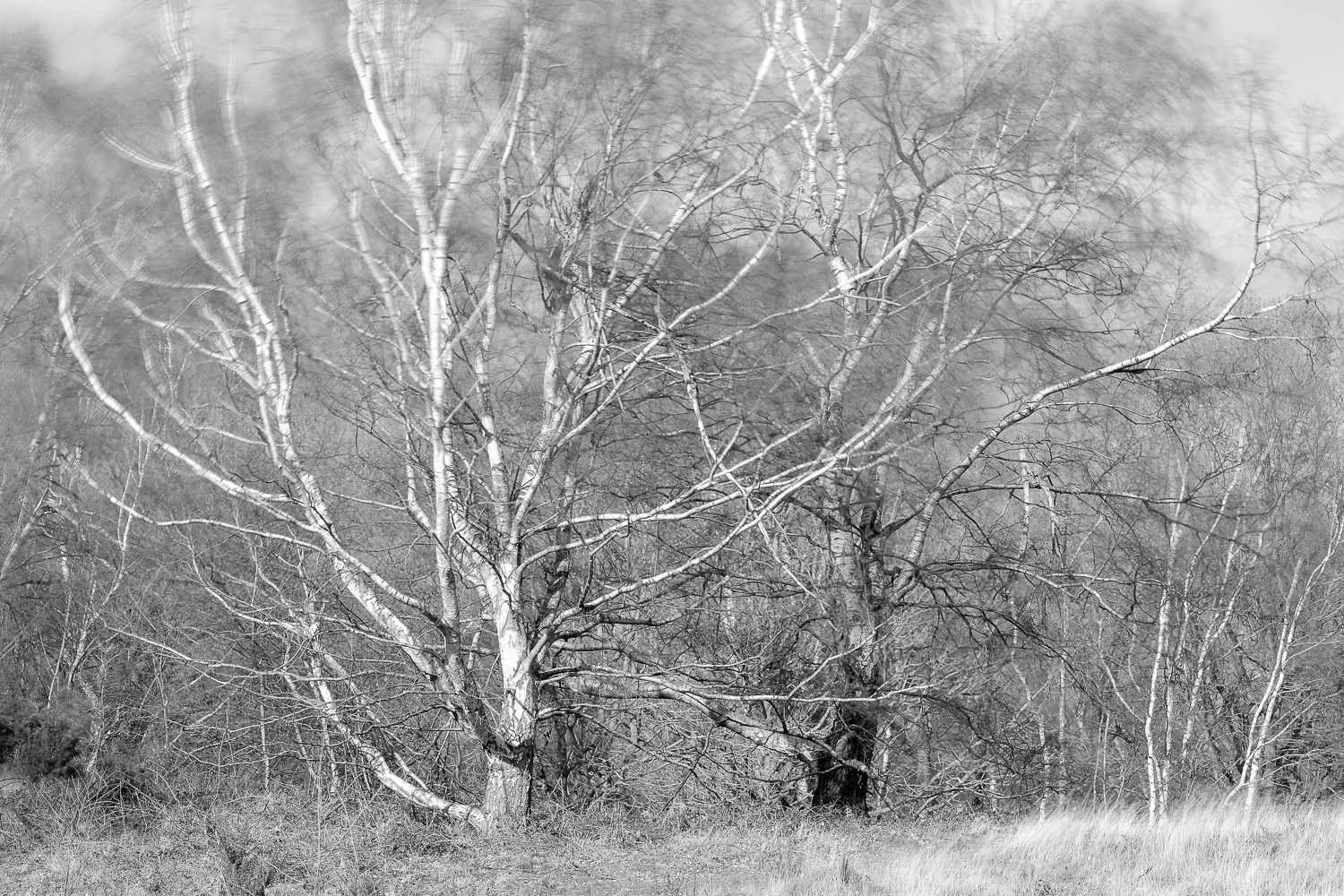S i l v e r   B i r c h e s  -  S h e r w o o d   H e a t h.

S c i r y u d a.   S h e r w o o d   F o r e s t,   N o t t i n g h a m s h i r e.

Sciryuda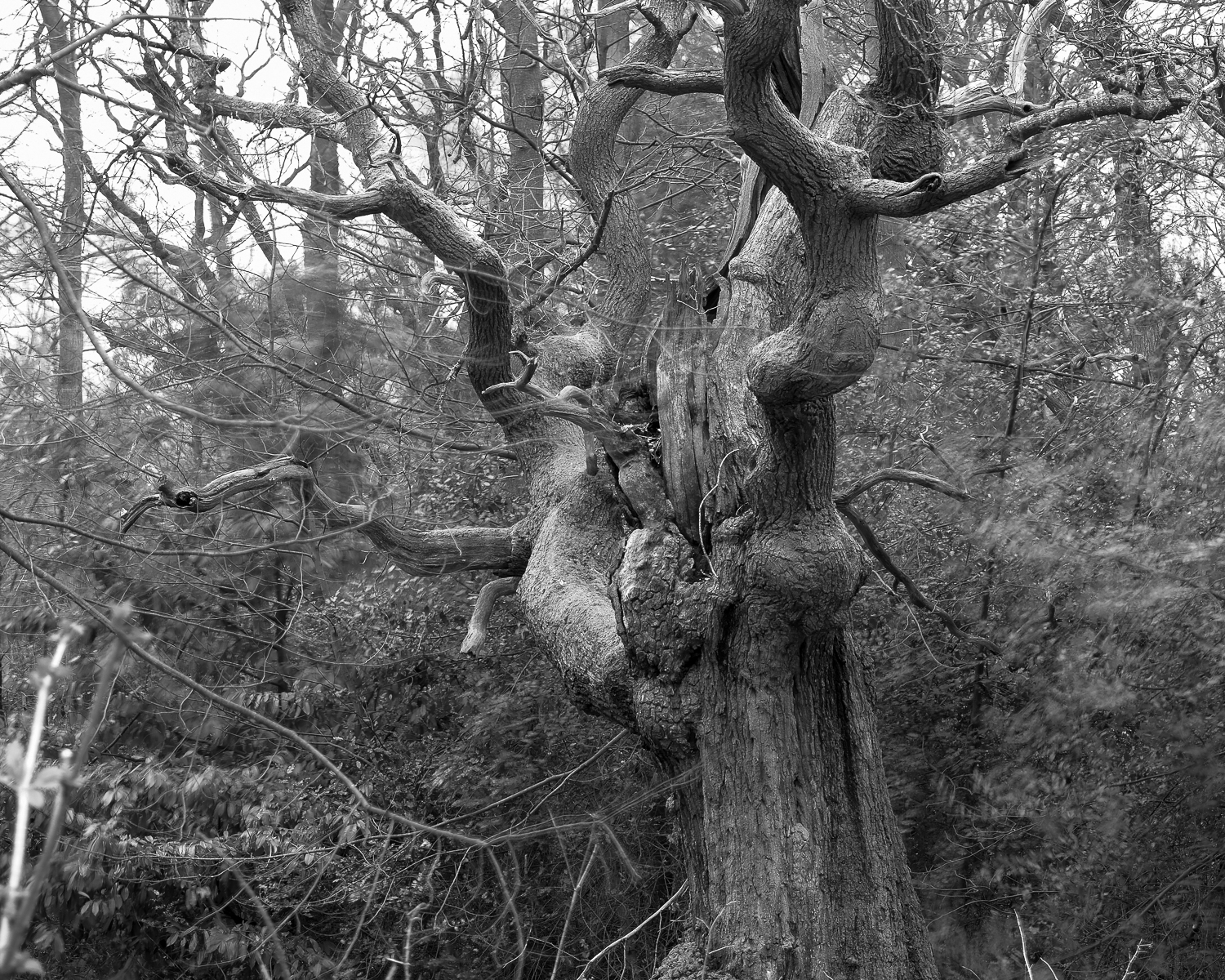A n c i e n t   O a k   -   C a b i n   P l a n t a t i o n

S c i r y u d a,   S h e r w o o d   F o r e s t,   N o t t i n g h a m s h i r e.

Sciryuda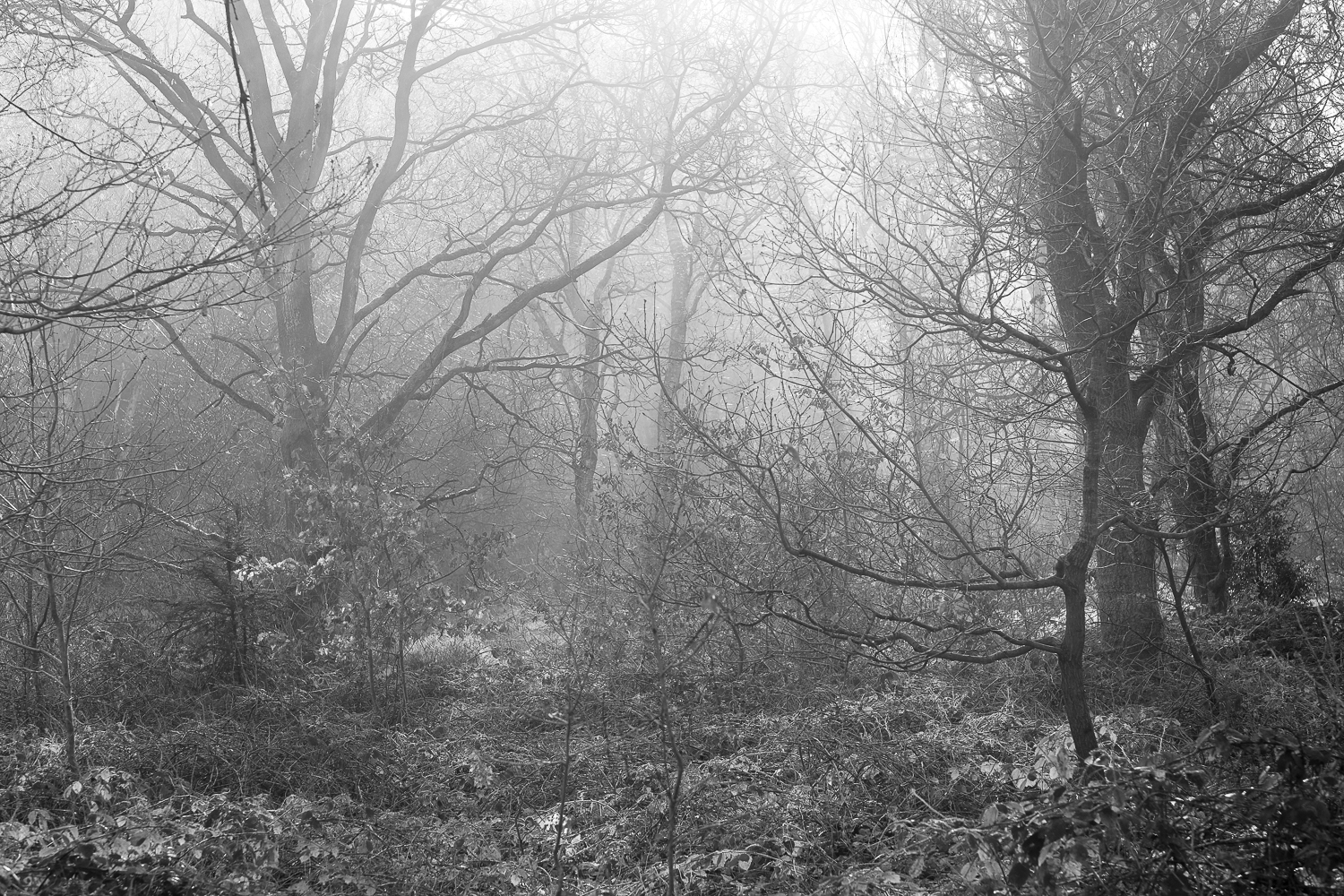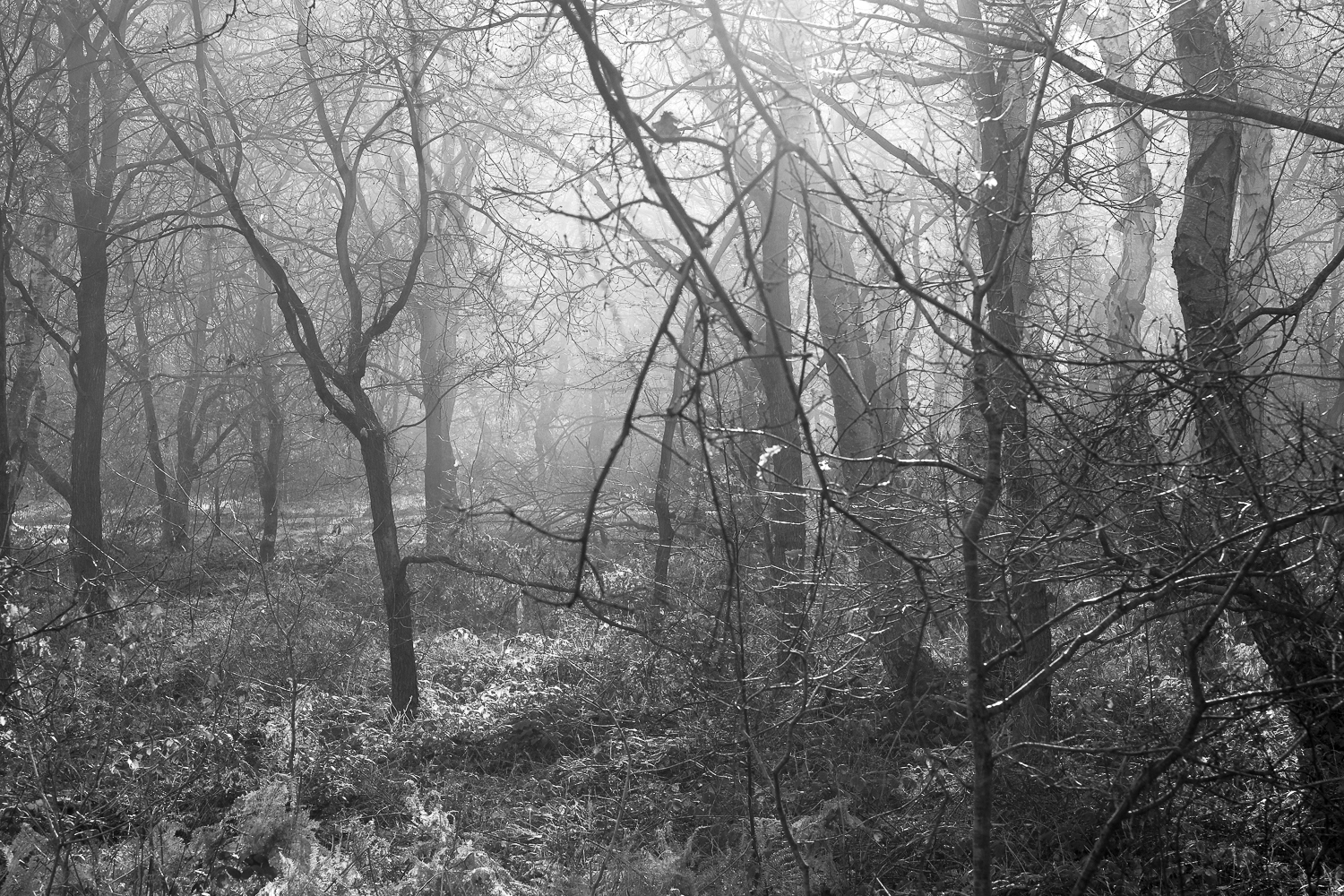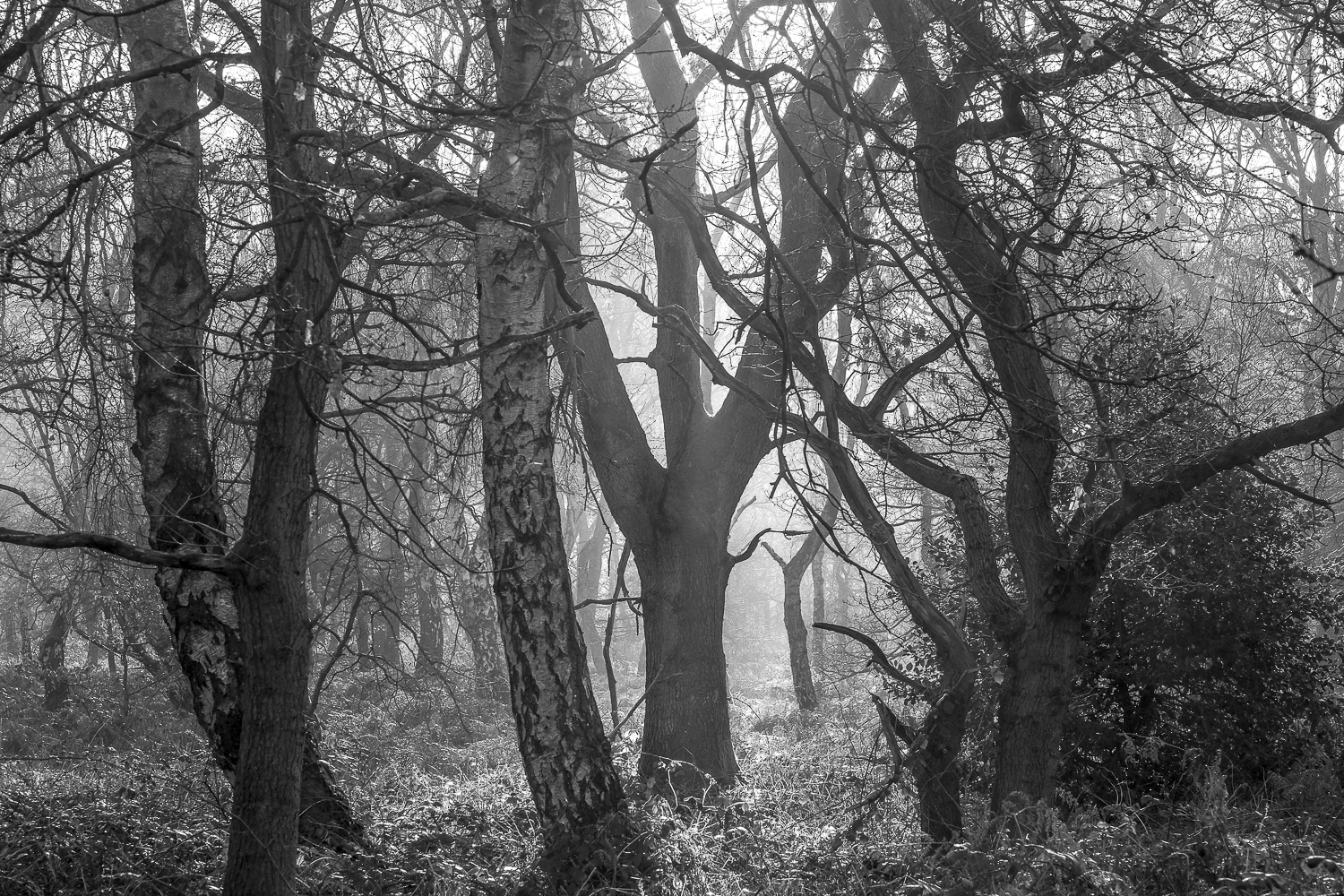Q u e e n   O a k   P l a n t a t i o n.

S c i r y u d a,   S h e r w o o d   F o r e s t,   N o t t i n g h a m s h i r e.

Sciryuda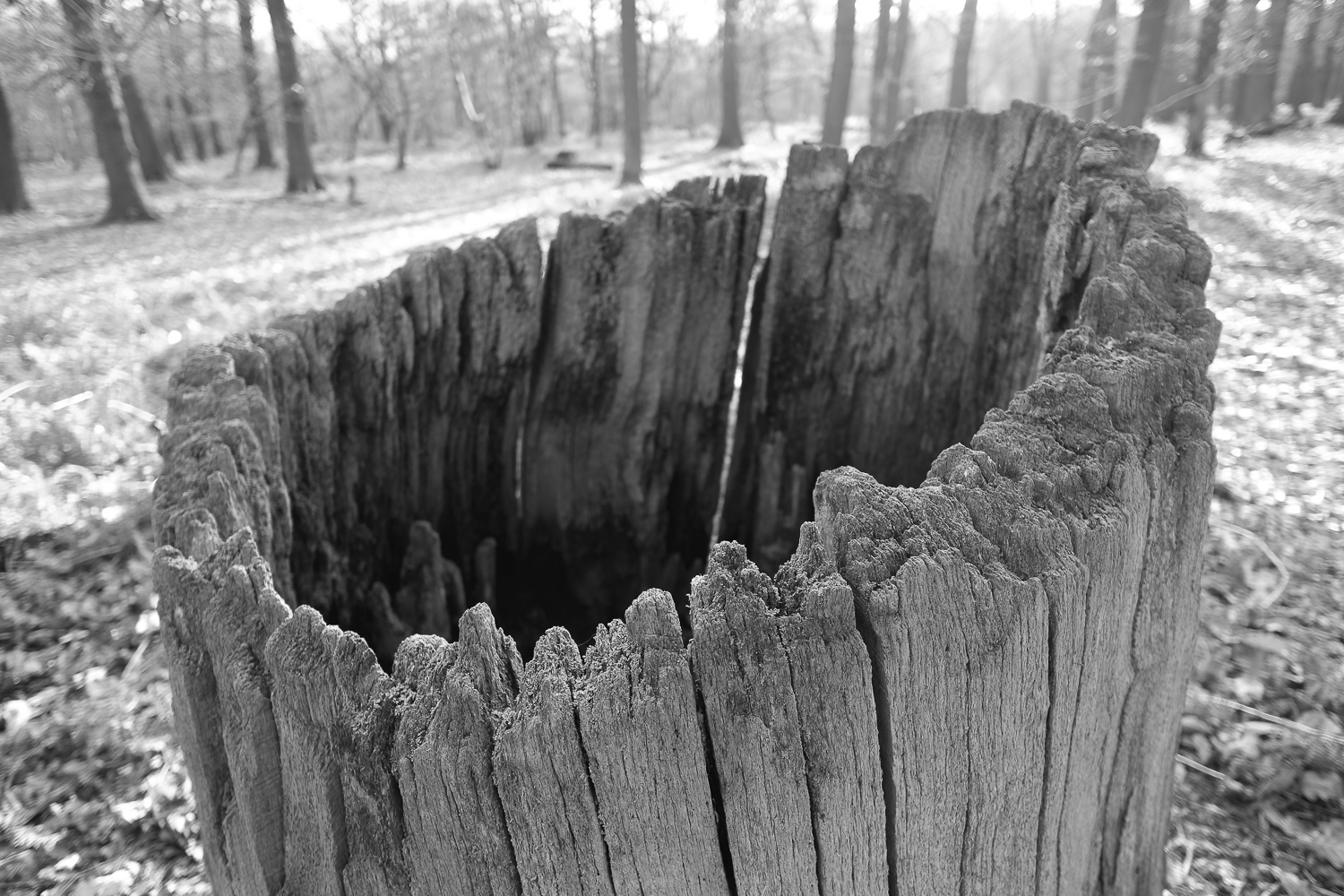S a w n   S t u m p   ( A n c i e n t   O a k )  -  B i r k l a n d s

S c i r y u d a.   S h e r w o o d   F o r e s t,   N o t t i n g h a m s h i r e.